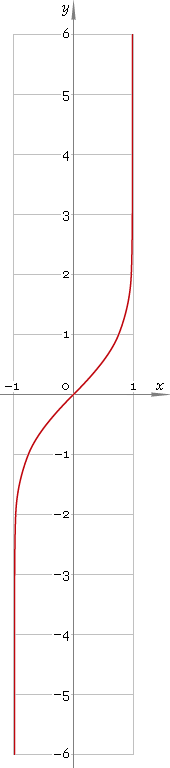The Art of Interface

# artanh or arth — arc-hyperbolic tangent function

Category. Mathematics.

Abstract. Arc-hyperbolic tangent: definition, plot, properties and identities.

## 1. Definition

Arc-hyperbolic tangent is inverse of hyperbolic tangent function. With the help of natural logarithm it can be represented as:

artanhx ≡ ln[(1 + x) /(1 − x)] /2

## 2. Plot

Arc-hyperbolic tangent is antisymmetric function defined in the range (−1, 1), points x = ±1 are singular ones. Its plot is depicted below — fig. 1.Fig. 1. Plot of the arc-hyperbolic tangent function y = artanhx.

Function codomain is entire real axis.

## 3. Identities

Property of antisymmetry:

artanh−x = −artanhx

Reciprocal argument:

artanh(1/x) = arcothx

Sum and difference:

artanhx + artanhy = artanh[(x + y) /(1 + xy)]
artanhx − artanhy = artanh[(xy) /(1 − xy)]

## 4. Support

Arc-hyperbolic tangent function artanh or arth of the real argument is supported by free version of the Librow calculator.

Arc-hyperbolic tangent function artanh or arth of the complex argument is supported by professional version of the Librow calculator.

## 5. How to use

To calculate arc-hyperbolic tangent of the number:

``artanh(-.5);``

To calculate arc-hyperbolic tangent of the current result:

``artanh(rslt);``

To calculate arc-hyperbolic tangent of the number x in memory:

``artanh(mem[x]);``